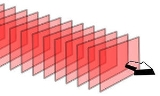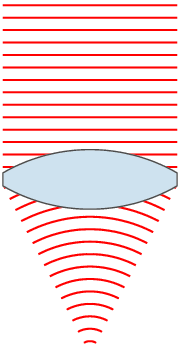xWavefrontEncyclopedia
In physics
Physics
Physics is a natural science that involves the study of matter and its motion through spacetime, along with related concepts such as energy and force. More broadly, it is the general analysis of nature, conducted in order to understand how the universe behaves.Physics is one of the oldest academic...

, a wavefront is the locus
Locus (mathematics)
In geometry, a locus is a collection of points which share a property. For example a circle may be defined as the locus of points in a plane at a fixed distance from a given point....

(a line
Line (mathematics)
The notion of line or straight line was introduced by the ancient mathematicians to represent straight objects with negligible width and depth. Lines are an idealization of such objects...

, or, in a wave
Wave
In physics, a wave is a disturbance that travels through space and time, accompanied by the transfer of energy.Waves travel and the wave motion transfers energy from one point to another, often with no permanent displacement of the particles of the medium—that is, with little or no associated mass...

propagating in 3 dimensions, a surface
Surface
In mathematics, specifically in topology, a surface is a two-dimensional topological manifold. The most familiar examples are those that arise as the boundaries of solid objects in ordinary three-dimensional Euclidean space R3 — for example, the surface of a ball...

) of point
Point (geometry)
In geometry, topology and related branches of mathematics a spatial point is a primitive notion upon which other concepts may be defined. In geometry, points are zero-dimensional; i.e., they do not have volume, area, length, or any other higher-dimensional analogue. In branches of mathematics...

s having the same phase
Phase (waves)
Phase in waves is the fraction of a wave cycle which has elapsed relative to an arbitrary point.-Formula:The phase of an oscillation or wave refers to a sinusoidal function such as the following:...

. Since infrared, optical, x-ray and gamma-ray frequencies are so high, the temporal component of electromagnetic waves is usually ignored at these wavelengths, and it is only the phase of the spatial oscillation that is described. Additionally, most optical systems and detectors are indifferent to polarization, so this property of the wave is also usually ignored. At radio wavelengths, the polarization becomes more important, and receivers are usually phase-sensitive. Many audio detectors are also phase-sensitive.## Simple wavefronts and propagation

Optical systems can be described with Maxwell's equations
Maxwell's equations
Maxwell's equations are a set of partial differential equations that, together with the Lorentz force law, form the foundation of classical electrodynamics, classical optics, and electric circuits. These fields in turn underlie modern electrical and communications technologies.Maxwell's equations...

, and linear propagating waves such as sound or electron beams have similar wave equations. However, given the above simplifications, Huygens' principle provides a quick method to predict the propagation of a wavefront through, for example, free space. The construction is as follows: Let every point on the wavefront be considered a new point source
Point source
A point source is a localised, relatively small source of something.Point source may also refer to:*Point source , a localised source of pollution**Point source water pollution, water pollution with a localized source...

. By calculating the total effect from every point source, the resulting field at new points can be computed. Computational algorithms are often based on this approach. Specific cases for simple wavefronts can be computed directly. For example, a spherical wavefront will remain spherical as the energy of the wave is carried away equally in all directions. Such directions of energy flow, which are always perpendicular to the wavefront, are called rays creating multiple wavefronts.

The simplest form of a wavefront is the plane wave
Plane wave
In the physics of wave propagation, a plane wave is a constant-frequency wave whose wavefronts are infinite parallel planes of constant peak-to-peak amplitude normal to the phase velocity vector....

, where the rays are parallel
Parallel (geometry)
Parallelism is a term in geometry and in everyday life that refers to a property in Euclidean space of two or more lines or planes, or a combination of these. The assumed existence and properties of parallel lines are the basis of Euclid's parallel postulate. Two lines in a plane that do not...

to one-another. The light from this type of wave is referred to as collimated light. The plane wavefront is a good model for a surface-section of a very large spherical wavefront; for instance, sunlight strikes the earth with a spherical wavefront that has a radius of about 150 million kilometers (1 AU
Astronomical unit
An astronomical unit is a unit of length equal to about or approximately the mean Earth–Sun distance....

). For many purposes, such a wavefront can be considered planar.

## Wavefront aberrations

Methods utilizing wavefront measurements or predictions can be considered an advanced approach to lens optics, where a single focal distance may not exist due to lens thickness or imperfections. Note also that for manufacturing reasons, a perfect lens has a spherical (or toroidal) surface shape though, theoretically, the ideal surface would be aspheric. Shortcomings such as these in an optical system cause what are called optical aberrations
Aberration in optical systems
Aberrations are departures of the performance of an optical system from the predictions of paraxial optics. Aberration leads to blurring of the image produced by an image-forming optical system. It occurs when light from one point of an object after transmission through the system does not converge...

. The best-known aberrations include spherical aberration
Spherical aberration
thumb|right|Spherical aberration. A perfect lens focuses all incoming rays to a point on the [[Optical axis|optic axis]]. A real lens with spherical surfaces suffers from spherical aberration: it focuses rays more tightly if they enter it far from the optic axis than if they enter closer to the...

and coma
Coma (optics)
In optics , the coma in an optical system refers to aberration inherent to certain optical designs or due to imperfection in the lens or other components which results in off-axis point sources such as stars appearing distorted, appearing to have a tail like a comet...

.

However there may be more complex sources of aberrations such as in a large telescope due to spatial variations in the index of refraction of the atmosphere. The deviation of a wavefront in an optical system from a desired perfect planar wavefront is called the wavefront aberration. Wavefront aberrations are usually described as either a sampled image or a collection of two-dimensional polynomial terms. Minimization of these aberrations is considered desirable for many applications in optical systems.

## Wavefront sensor and reconstruction techniques

A wavefront sensor
Wavefront sensor
A wavefront sensor is a device for measuring the aberrations of an optical wavefront. Although an amplitude splitting interferometer such as the Michelson interferometer could be called a wavefront sensor, the term is normally applied to instruments that do not require an unaberrated reference...

is a device which measures the wavefront aberration in a coherent signal to describe the optical quality or lack thereof in an optical system. A very common method is to use a Shack-Hartmann
Shack-Hartmann
A Shack–Hartmann wavefront sensor is an optical instrument used to characterize an imaging system. It is a wavefront sensor commonly used in adaptive optics systems. It consists of an array of lenses of the same focal length. Each is focused onto a photon sensor...

lenslet
Lenslet
A lenslet is literally a small lens. The fact that distinguishes it from a small lens is that it is part of a lenslet array. A lenslet array consists of a set of lenslets in the same plane. Each lenslet normally has the same focal length....

array. There are many applications that include adaptive optics
Adaptive optics is a technology used to improve the performance of optical systems by reducing the effect of wavefront distortions. It is used in astronomical telescopes and laser communication systems to remove the effects of atmospheric distortion, and in retinal imaging systems to reduce the...

, optical metrology and even the measurement of the aberrations in the eye
Human eye
The human eye is an organ which reacts to light for several purposes. As a conscious sense organ, the eye allows vision. Rod and cone cells in the retina allow conscious light perception and vision including color differentiation and the perception of depth...

itself. In this approach, a weak laser source is directed into the eye and the reflection off the retina
Retina
The vertebrate retina is a light-sensitive tissue lining the inner surface of the eye. The optics of the eye create an image of the visual world on the retina, which serves much the same function as the film in a camera. Light striking the retina initiates a cascade of chemical and electrical...

is sampled and processed.

Alternative wavefront sensing techniques to the Shack-Hartmann
Shack-Hartmann
A Shack–Hartmann wavefront sensor is an optical instrument used to characterize an imaging system. It is a wavefront sensor commonly used in adaptive optics systems. It consists of an array of lenses of the same focal length. Each is focused onto a photon sensor...

system are emerging. Mathematical techniques like phase imaging or curvature sensing are also capable of providing wavefront estimations. These algorithms compute wavefront images from conventional brightfield images at different focal planes without the need for specialised wavefront optics. While Shack-Hartmann lenslet arrays are limited in lateral resolution to the size of the lenslet array, techniques such as these are only limited by the resolution of digital images used to compute the wavefront measurements.

Another application of software reconstruction of the phase is the control of telescopes through the use of adaptive optics. A common method is the Roddier test, also called wavefront curvature sensing. It yields good correction, but needs an already good system as a starting point.

• Huygens' principle
• Wavefront sensor
Wavefront sensor
A wavefront sensor is a device for measuring the aberrations of an optical wavefront. Although an amplitude splitting interferometer such as the Michelson interferometer could be called a wavefront sensor, the term is normally applied to instruments that do not require an unaberrated reference...

Adaptive optics is a technology used to improve the performance of optical systems by reducing the effect of wavefront distortions. It is used in astronomical telescopes and laser communication systems to remove the effects of atmospheric distortion, and in retinal imaging systems to reduce the...

• Deformable mirror
Deformable mirror
Deformable mirror represents the most convenient tool for wavefront control and correction of optical aberrations. Deformable mirrors are used in combination with wavefront sensors and real-time control systems in adaptive optics...